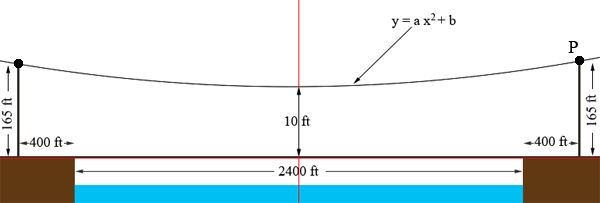SEARCH HOMEMath Central Quandaries & QueriesQuestion from jennifer, a student: suspension bridges like the golden gate bridge, are used to span large distances. when the main curved cables are attached to the deck by vertical cables they will end up in the shape of parabola. assume that we need to build a bridge that spans 2,400 feet. the two towers 165feet tall each where placed at 400feet from either side. the lowest point of the center of the bridge at 10feet. vertical suspension cables where placed at 25foot interval. how many feet of cable are needed to connect the deck to the main cables between the two towers? show all working.Hi Jennifer,

I drew a rough diagram (not so scale), put some dimensions on it and a coordinate system in red.The origin is at the road surface directly below the lowest point on the cable so the equation of the parabola is $y = a x^2 + b.$

When $x = 0$ what is the value of $y?$ What is the value of $b?$

What are the coordinates of the point P? Substitute into the equation. What is the value of $a?$

Now that you know the equation of the parabola you should be able to complete the problem.

PennyMath Central is supported by the University of Regina and The Pacific Institute for the Mathematical Sciences.